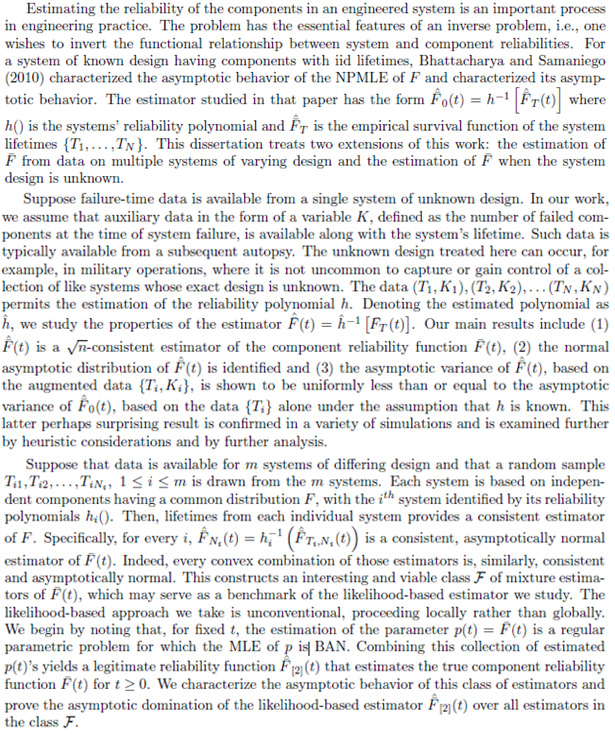# PhD Dissertation Abstracts: 2013

## Statistics PhD Alumni 2013:Chris Dienes Yin Jin Kimihiro Noguchi Wanli Qiao Ru Wang

### ﻿Christopher Dienes (2013)

TITLE: On-line monitoring in linear time series models

ABSTRACT: Linear time series models have been instrumental in the analysis of time dependent data and have applications in many areas of scientific interest. In order to perform model estimation and produce reliable forecasts, practitioners require model structure and specifications to remain constant over time. However, assumptions of model stationarity are often violated in observed data. This motivates the use of statistical testing procedures developed for detection of structural breaks. This talk introduces several new on-line procedures which sequentially test for model stability as new data become available. The proposed methodology does not make the unrealistic assumption of known initial parameters which is commonly seen in the sequential literature. Rather, the approach incorporates an estimation step based on non-contaminated training data prior to the start of monitoring. Both asymptotic and finite sample properties are addressed through theory and simulations. The methodology is applied to an environmental science data set. The last part of the talk will address the limiting distributions of stopping times. Special consideration is given to breaks in level and scale. Results indicate the precise form of the asymptotic depends both on the location of the break point and the size of the change implied by an induced drift term. The theoretical results are accompanied by a simulation study and application.

### ﻿Yin Jin (2013)

TITLE: Estimating Component Reliability Using System Lifetime Data

ABSTRACT: (Note: has statistical symbols that could not be reproduced in text format, so a jpg of the text is provided)### ﻿Kimihiro Noguchi (2013)

TITLE: Exploratory Analysis and Modeling of Financial Time Series

ABSTRACT: Novel joint semiparametric spline-based modeling of conditional mean and volatility of financial time series is proposed and evaluated on recent daily stock return data. The new modeling approach incorporates functions of lagged response variables and time as predictors. The conditional mean model is additive. The conditional volatility model is multiplicative and linearized with a logarithmic transformation. In addition, a cube-root power transformation is employed to symmetrize the lagged absolute fluctuations. Using cubic splines, the model can be written as a multiple linear regression, thereby allowing predictions to be obtained in a simple manner. Reliable estimation of the model parameters is achieved by trimmed least squares (TLS) estimation for which a reasonable amount of trimming is suggested. A new model selection criterion corresponding to TLS is also derived. Moreover, the (three-parameter) generalized gamma distribution is identified as suitable for the absolute multiplicative errors and shown to work well for predictions and also for the calculation of quantiles, which is important to determine the value-at-risk. Further, trading volume and leverage effect are considered as predictors for the conditional volatility model. Then, the prediction performance is compared to the classical GARCH and APGARCH models. The results suggest that the proposed model may possess a high predictive power for future conditional volatility. Lastly, the rate of convergence for the relevant B-spline model is derived.

### ﻿Wanli Qiao (2013)

TITLE: On Estimation of Filamentary Structures

ABSTRACT: A filamentary structure is defined as a lower-dimensional manifold consisting of local modes in direction orthogonal to the manifold itself. These structures are mostly present in medical imaging, remote sensing, seismology and astronomy. A substantial amount of literature has tried to develop algorithms to estimate these geometric structures. However, explicit mathematical definitions are rarely provided to describe them and hence statistical inference of their estimation is not common.

This dissertation aims to establish nonparametric statistical analysis of filamentary structures, where we focus on the 2-dimensional Euclidean space. We propose an estimator based on integral curves and derive uniform consistency and convergence rates of this estimator. In order to assess the reliability of the estimation, we study the asymptotic probability distribution of the maximal deviation of the estimator from the ground truth. The derivation of the asymptotic distribution can be solved with the help of a limit result on the maximal deviation of a sequence of non-stationary Gaussian fields on a growing manifold. Two algorithms for calculating filament estimators will be presented, with one of them derived from the studied filament estimator and the other one being motivated by some more empirical considerations. The performance of these algorithms is illustrated by an application to a galaxy dataset from an astronomical study.